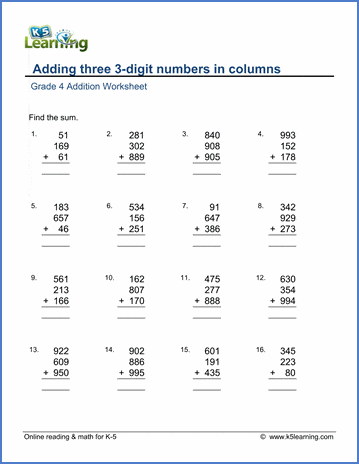Example

Add 2-digit numbers (no carrying)   22 + 21 =
Add 2-digit numbers (sum under 100)   53 + 19 =
Add 2-digit numbers   46 + 89 =
5 + 40 + 55 =

40 + 40 + 30 + 30 =
Add whole hundreds   300 + 500 =
100 + 200 + 300 =

Complete whole hundreds (missing addend)   ___ + 606 = 900
Complete whole thousands (missing addend)   ___ + 8,700 = 9,000

Add a 3-digit number and a 1-digit number   295 + 8 =
Add three 2-digit numbers   39 + 11 +16 =

Missing addend   20 + ___ = 34
Missing addend - harder   80 + ___ = 743

367

+ 678

123

+ 345

+ 567

345

+ 678

+ 789

+ 890

123

+ 345

+ 567

+ 789

+ 890

1,234

+ 2,345

1,234

+ 2,345

+ 4,567

1,234

+ 2,345

+ 4,567

+ 7,890

1,234

+ 2,345

+ 3,456
+ 5,678

+ 6,789

98,779

+ 12,331

945,687

+ 768,989

+ 368,966

467,870

+ 800,760

+ 766,469

+ 355,689

Mixed add & subtract word problems   Word problems
Mixed 4 operations word problems   Word problems